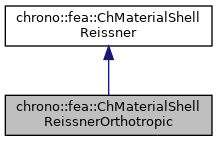chrono::fea::ChMaterialShellReissnerOrthotropic Class Reference

## Description

Material definition.

This class implements material properties for a layer from the Reissner theory, for the case of orthotropic linear elastic material. This is useful for laminated shells. One direction can be made softer than the other. Note that the angle and the thickness are defined when adding this material to a finite element as a layer.

#include <ChMaterialShellReissner.h>

Inheritance diagram for chrono::fea::ChMaterialShellReissnerOrthotropic:[legend]
Collaboration diagram for chrono::fea::ChMaterialShellReissnerOrthotropic:[legend]

## Public Member Functions

ChMaterialShellReissnerOrthotropic (double m_rho, double m_E_x, double m_E_y, double m_nu_xy, double m_G_xy, double m_G_xz, double m_G_yz, double m_alpha=1.0, double m_beta=0.1)
Construct an orthotropic material. More...

ChMaterialShellReissnerOrthotropic (double m_rho, double m_E, double m_nu, double m_alpha=1.0, double m_beta=0.1)
Construct an orthotropic material as sub case isotropic. More...

double Get_E_x () const
Return the elasticity moduli, on x.

double Get_E_y () const
Return the elasticity moduli, on y.

double Get_nu_xy () const
Return the Poisson ratio, for xy.

double Get_nu_yx () const
Return the Poisson ratio, for yx (follows xy as it must be nu_yx*E_x = nu_xy*E_y)

double Get_G_xy () const
Return the shear mod, in plane.

double Get_G_xz () const
Return the shear mod, transverse.

double Get_G_yz () const
Return the shear mod, transverse.

double Get_alpha () const
Return the shear factor.

double Get_beta () const
Return the torque factor.

virtual void ComputeStress (ChVector<> &n_u, ChVector<> &n_v, ChVector<> &m_u, ChVector<> &m_v, const ChVector<> &eps_u, const ChVector<> &eps_v, const ChVector<> &kur_u, const ChVector<> &kur_v, const double z_inf, const double z_sup, const double angle)
The FE code will evaluate this function to compute per-unit-length forces/torques given the u,v strains/curvatures. More...

virtual void ComputeTangentC (ChMatrixRef mC, const ChVector<> &eps_u, const ChVector<> &eps_v, const ChVector<> &kur_u, const ChVector<> &kur_v, const double z_inf, const double z_sup, const double angle)
Compute [C] , that is [ds/de], the tangent of the constitutive relation per-unit-length forces/torques vs strains. More...Public Member Functions inherited from chrono::fea::ChMaterialShellReissner
ChMaterialShellReissner ()
Construct an isotropic material.

double Get_rho () const
Return the material density.Protected Attributes inherited from chrono::fea::ChMaterialShellReissner
double m_rho
density

## Constructor & Destructor Documentation

 chrono::fea::ChMaterialShellReissnerOrthotropic::ChMaterialShellReissnerOrthotropic ( double m_rho, double m_E_x, double m_E_y, double m_nu_xy, double m_G_xy, double m_G_xz, double m_G_yz, double m_alpha = 1.0, double m_beta = 0.1 )

Construct an orthotropic material.

Parameters
 m_rho material density m_E_x Young's modulus on x m_E_y Young's modulus on y m_nu_xy Poisson ratio xy (for yx it holds: nu_yx*E_x = nu_xy*E_y) m_G_xy Shear modulus, in plane m_G_xz Shear modulus, transverse m_G_yz Shear modulus, transverse m_alpha shear factor m_beta torque factor
 chrono::fea::ChMaterialShellReissnerOrthotropic::ChMaterialShellReissnerOrthotropic ( double m_rho, double m_E, double m_nu, double m_alpha = 1.0, double m_beta = 0.1 )

Construct an orthotropic material as sub case isotropic.

Parameters
 m_rho material density m_E Young's modulus on x m_nu Poisson ratio m_alpha shear factor m_beta torque factor

## Member Function Documentation

 void chrono::fea::ChMaterialShellReissnerOrthotropic::ComputeStress ( ChVector<> & n_u, ChVector<> & n_v, ChVector<> & m_u, ChVector<> & m_v, const ChVector<> & eps_u, const ChVector<> & eps_v, const ChVector<> & kur_u, const ChVector<> & kur_v, const double z_inf, const double z_sup, const double angle )
virtual

The FE code will evaluate this function to compute per-unit-length forces/torques given the u,v strains/curvatures.

Parameters
 n_u forces along u direction (per unit length) n_v forces along v direction (per unit length) m_u torques along u direction (per unit length) m_v torques along v direction (per unit length) eps_u strains along u direction eps_v strains along v direction kur_u curvature along u direction kur_v curvature along v direction z_inf layer lower z value (along thickness coord) z_sup layer upper z value (along thickness coord) angle layer angle respect to x (if needed) -not used in this, isotropic

Implements chrono::fea::ChMaterialShellReissner.

 void chrono::fea::ChMaterialShellReissnerOrthotropic::ComputeTangentC ( ChMatrixRef mC, const ChVector<> & eps_u, const ChVector<> & eps_v, const ChVector<> & kur_u, const ChVector<> & kur_v, const double z_inf, const double z_sup, const double angle )
virtual

Compute [C] , that is [ds/de], the tangent of the constitutive relation per-unit-length forces/torques vs strains.

Parameters
 mC tangent matrix eps_u strains along u direction eps_v strains along v direction kur_u curvature along u direction kur_v curvature along v direction z_inf layer lower z value (along thickness coord) z_sup layer upper z value (along thickness coord) angle layer angle respect to x (if needed) -not used in this, isotropic

Reimplemented from chrono::fea::ChMaterialShellReissner.